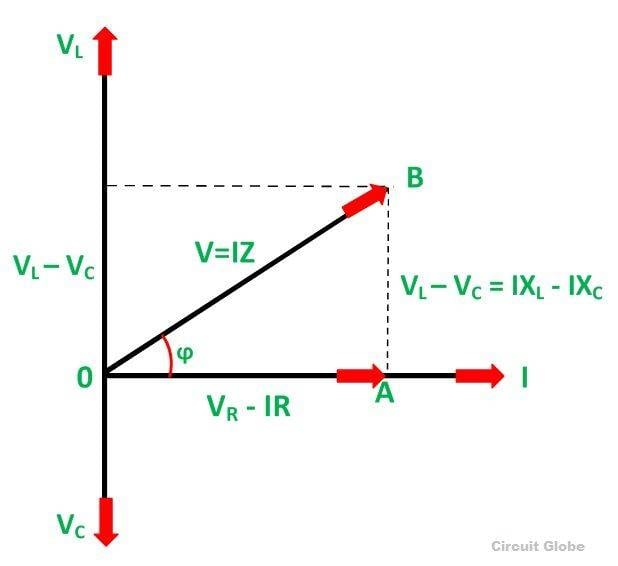# Calculate Real and Reactive Power and write equation for S = P + jQ

## Homework Statement

v = 141.4sin(wt + 15)
i = 14.14 cos(wt - 45)

## Homework Equations

Draw Phasors.
P = VI cos(theta)
Q = VI sin(theta)
S = P + jQ
theta is angle between V and I

## The Attempt at a Solution

[/B]
V = 141.4sin(wt + 15)
So Vrms = 141.4/1.414 sin(wt + 15)
= 100 sin (wt + 15)

I = 14.14 cos(wt - 45)
Now cos (-theta) = cos(theta)
So I = 14.14 cos (45-wt)
So I = 14.14 sin(90-45 + wt)
So I = 14.14 sin(45 + wt)
I rms = 10sin(wt + 45)

Now Vrms = 100sin(wt + 15)
I rms = 10sin(wt + 45)
I leads V by 30 degrees.
theta = 30 degrees.
P = VI cos(theta) = 100 * 10 * cos(30) = 866W
Q = VI sin(theta) = 100 * 10 * sin(30) = 500VAR
So S = P + jQ = 866 + j500

But in answer it is given S = 866 - j500

Where does the minus sign come from?
I understand that it is leading power factor so it gives Q but mathematically sin(30) is positive value. So why should S have negative VAR?

scottdave
Homework Helper
Leading current (current leads voltage) gives phasors pointing down (negative angle). Lagging current (current lags voltage) give phasors pointing up (positive angle).
That is the convention.#### Attachments

•jaus tail
cnh1995
Homework Helper
Gold Member

## Homework Statement

v = 141.4sin(wt + 15)
i = 14.14 cos(wt - 45)

## Homework Equations

Draw Phasors.
P = VI cos(theta)
Q = VI sin(theta)
S = P + jQ
theta is angle between V and I

## The Attempt at a Solution

[/B]
V = 141.4sin(wt + 15)
So Vrms = 141.4/1.414 sin(wt + 15)
= 100 sin (wt + 15)

I = 14.14 cos(wt - 45)
Now cos (-theta) = cos(theta)
So I = 14.14 cos (45-wt)
So I = 14.14 sin(90-45 + wt)
So I = 14.14 sin(45 + wt)
I rms = 10sin(wt + 45)

Now Vrms = 100sin(wt + 15)
I rms = 10sin(wt + 45)
I leads V by 30 degrees.
theta = 30 degrees.
P = VI cos(theta) = 100 * 10 * cos(30) = 866W
Q = VI sin(theta) = 100 * 10 * sin(30) = 500VAR
So S = P + jQ = 866 + j500

But in answer it is given S = 866 - j500

Where does the minus sign come from?
I understand that it is leading power factor so it gives Q but mathematically sin(30) is positive value. So why should S have negative VAR?
By convention, leading VARs are negative and lagging VARs are positive, and S=VI*.
(If it were the opposite, you'd have to use S=IV*.)

•jaus tail
So mathematically when we calculate Q then it is V multiplied by I multiplied by the angle by which the voltage leads the current.
Thus here we'll get -theta.

cnh1995
Homework Helper
Gold Member
Take for example, V=20∠30° and I=4∠-30°.
Here, the current is lagging and by the convention S=VI*, you'll get S=P+jQ i.e. positive reactive power.

If you want lagging VARs to be negative (as your own convention), define S=IV*.